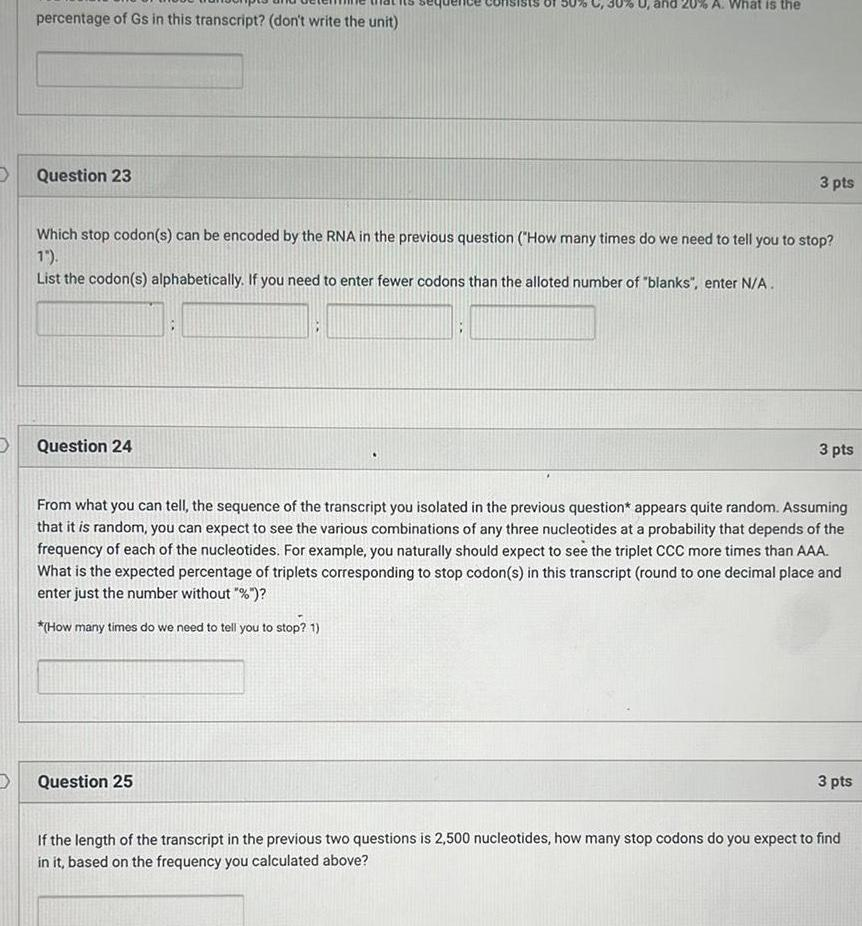Question:

# D percentage of Gs in this transcript don t write the unit

Last updated: 11/18/2023D percentage of Gs in this transcript don t write the unit Question 23 Question 24 U and 20 A What is the Which stop codon s can be encoded by the RNA in the previous question How many times do we need to tell you to stop 1 List the codon s alphabetically If you need to enter fewer codons than the alloted number of blanks enter N A 3 pts Question 25 3 pts From what you can tell the sequence of the transcript you isolated in the previous question appears quite random Assuming that it is random you can expect to see the various combinations of any three nucleotides at a probability that depends of the frequency of each of the nucleotides For example you naturally should expect to see the triplet CCC more times than AAA What is the expected percentage of triplets corresponding to stop codon s in this transcript round to one decimal place and enter just the number without How many times do we need to tell you to stop 1 3 pts If the length of the transcript in the previous two questions is 2 500 nucleotides how many stop codons do you expect to find in it based on the frequency you calculated above